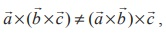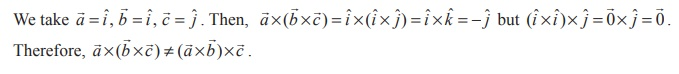Home | | Maths 12th Std | Vector triple product

# Vector triple product

Vector triple product is not associative.

Vector triple product

## Definition 6.5

For a given set of three vectors, the vector×(×) is called a  vector triple product.

Note

Given any three vectors,the following are vector triple products :Using the well known properties of the vector product, we get the following theorem.

## Theorem 6.7

The vector triple product satisfies the following properties.### Remark

Vector triple product is not associative. This means thatfor some vectors.

JustificationThe following theorem gives a simple formula to evaluate the vector triple product.

## Theorem 6.8 (Vector Triple product expansion)

For any three vectorswe haveProof

Let us choose the coordinate axes as follows :

Let x -axis be chosen along the line of action of, y -axis be chosen in the plane passing throughand parallel to, and z -axis be chosen perpendicular to the plane containingand. Then, we haveNote(3) In (×) ×c , consider the vectors inside the brackets, callas the middle vector andas the non-middle vector. Similarly, in,is the middle vector andis the non-middle vector. Then we observe that a vector triple product of these vectors is equal to

λ (middle vector) −µ (non-middle vector)

where λ is the dot product of the vectors other than the middle vector and μ is the dot

product of the vectors other than the non-middle vector.

Tags : Definition, Theorem, Proof , 12th Mathematics : UNIT 6 : Applications of Vector Algebra
Study Material, Lecturing Notes, Assignment, Reference, Wiki description explanation, brief detail
12th Mathematics : UNIT 6 : Applications of Vector Algebra : Vector triple product | Definition, Theorem, Proof